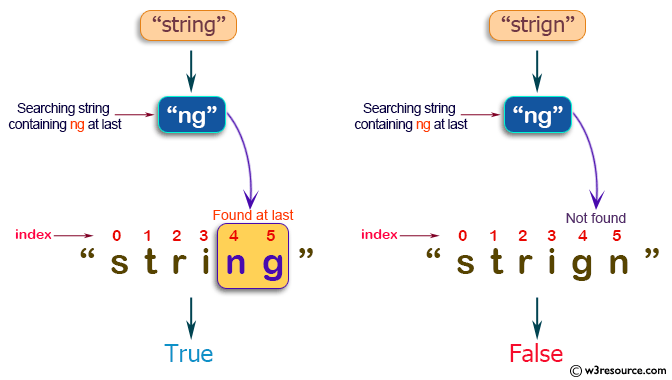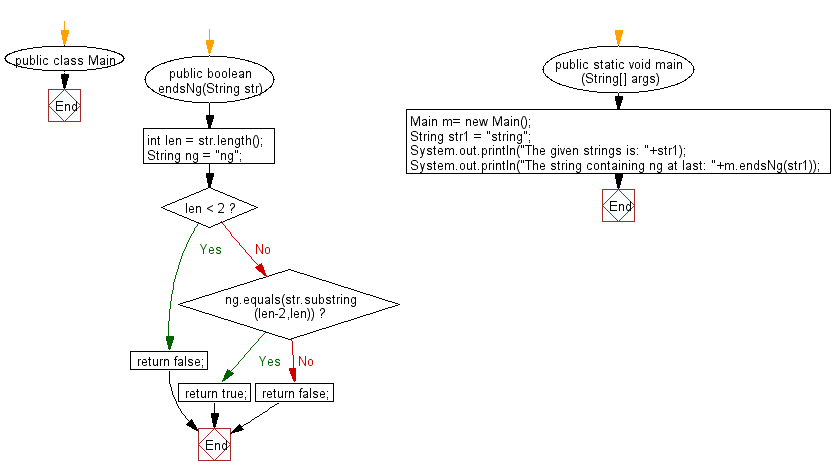﻿ Java exercises: Read a string and return true if it ends in 'ng' - w3resource# Java String Exercises: Read a string and return true if it ends in "ng"

## Java String: Exercise-58 with Solution

Write a Java program to read a string and return true if it ends in "ng".

Sample Solution:

Java Code:

``````import java.util.*;
public class Main
{
public boolean endsNg(String str)
{
int len = str.length();
String ng = "ng";
if (len < 2)
return false;
else if (ng.equals(str.substring(len-2,len)))
return true;
else
return false;
}
public static void main (String[] args)
{
Main m= new Main();
String str1 =  "string";
System.out.println("The given strings is: "+str1);
System.out.println("The string containing ng at last: "+m.endsNg(str1));
}
}
```
```

Sample Output:

```The given strings is: string
The string containing ng at last: true

The given strings is: strign
The string containing ng at last: false
```

Pictorial Presentation:Flowchart:Java Code Editor:

Improve this sample solution and post your code through Disqus

What is the difficulty level of this exercise?

﻿

New Content: Composer: Dependency manager for PHP, R Programming